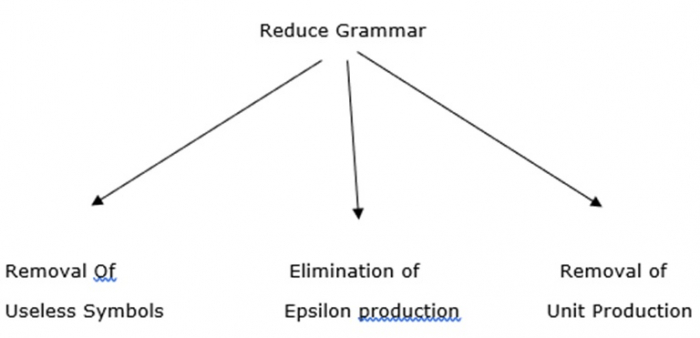# Explain the removal of useless symbols

All grammars are not always optimized, which means the grammar may consist of some extra symbols (non-terminals) which increase the length of grammar.

So, we have to reduce the grammar by removing such useless symbols.

## Properties

The properties to reduce grammar are explained below −

• Each non-terminal and terminal of G appears in the derivation of some word in L
• There should not be any production as X->Y where X and Y are non-terminals.
• If epsilon is not in language L then, there need not be in the production X-> ε.

The use of reducing grammar is as follows −## Definition

A symbol X is useful if there is a derivation of the form

S=>* aXb =* w

Otherwise, the symbol X is useless. Note that in a derivation, finally, we should get a string of terminals and all these symbols must be reachable from the start symbol S.

Those symbols and productions which are not at all used in the derivation are useless.

## Example

Consider the following example for removing symbol

S->aAa|bBb| ε
A->C|a
B->C|b
C->CDE| ε
D->A|B|ab

The useless symbols from the given grammar is E. Because E is not a derivative on the right hand side (RHS).

After removing the useless symbol, the production is as follows

S->aDa|bDb
D->a|b|ab